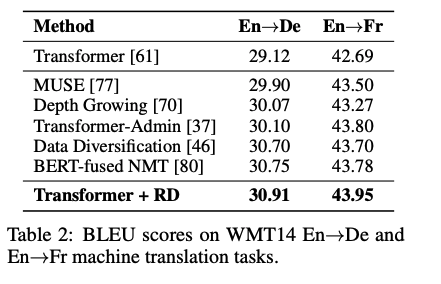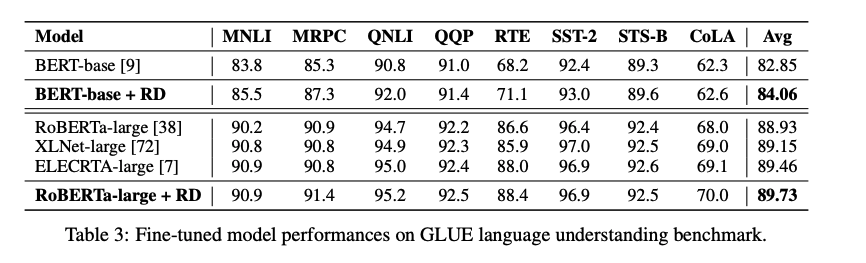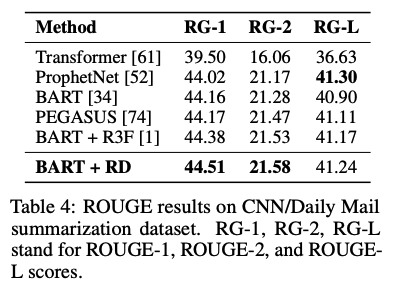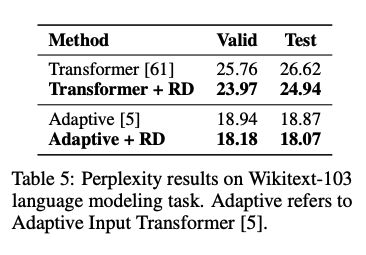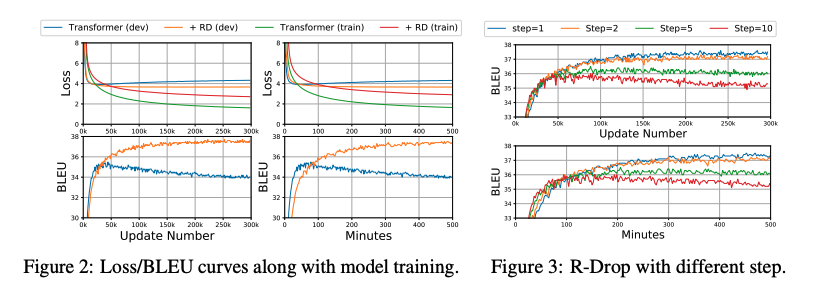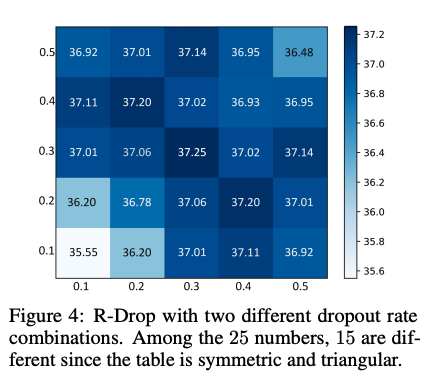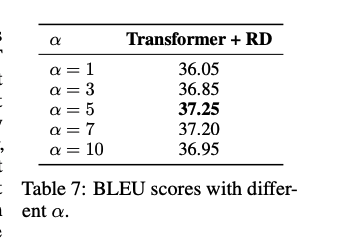## 方法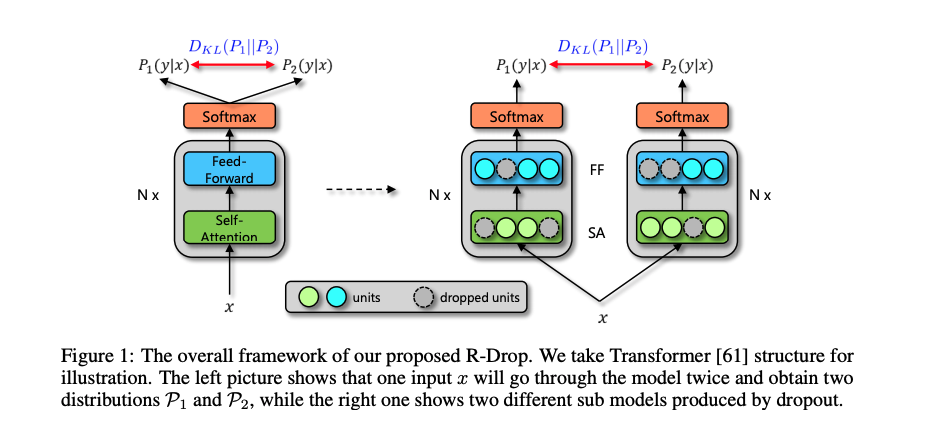$\mathcal{L}_{K L}^{i}=\frac{1}{2}\left(\mathcal{D}_{K L}\left(\mathcal{P}_{1}^{w}\left(y_{i} \mid x_{i}\right) \| \mathcal{P}_{2}^{w}\left(y_{i} \mid x_{i}\right)\right)+\mathcal{D}_{K L}\left(\mathcal{P}_{2}^{w}\left(y_{i} \mid x_{i}\right) \| \mathcal{P}_{1}^{w}\left(y_{i} \mid x_{i}\right)\right)\right)$

$\mathcal{L}_{N L L}^{i}=-\log \mathcal{P}_{1}^{w}\left(y_{i} \mid x_{i}\right)-\log \mathcal{P}_{2}^{w}\left(y_{i} \mid x_{i}\right)$

\begin{aligned} \mathcal{L}^{i}=\mathcal{L}_{N L L}^{i}+\alpha \cdot \mathcal{L}_{K L}^{i}=&-\log \mathcal{P}_{1}^{w}\left(y_{i} \mid x_{i}\right)-\log \mathcal{P}_{2}^{w}\left(y_{i} \mid x_{i}\right) \\ &+\frac{\alpha}{2}\left[\mathcal{D}_{K L}\left(\mathcal{P}_{1}^{w}\left(y_{i} \mid x_{i}\right) \| \mathcal{P}_{2}^{w}\left(y_{i} \mid x_{i}\right)\right)+\mathcal{D}_{K L}\left(\mathcal{P}_{2}^{w}\left(y_{i} \mid x_{i}\right) \| \mathcal{P}_{1}^{w}\left(y_{i} \mid x_{i}\right)\right)\right] \end{aligned}

## 实验

### 实验结果